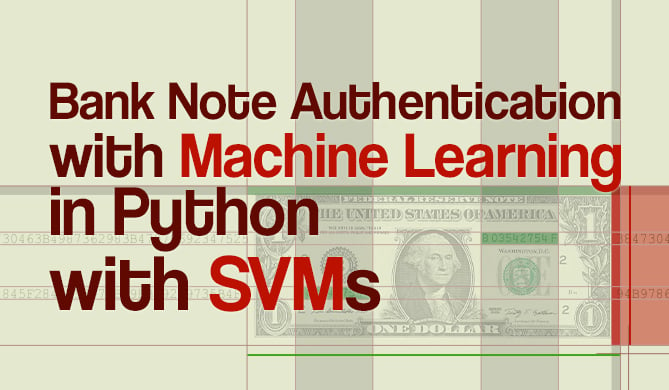Plot Decision Boundaries Using Python and Scikit-Learn

# Plot Decision Boundaries Using Python and Scikit-LearnTree-based models have become a popular choice for Machine Learning, not only due to their results, and the need for fewer transformations when working with data (due to robustness to input and scale invariance), but also because there is a way to take a peek inside of them to see what is going on with the data.

We'll assume you have just trained a tree-based classifier using a Decision Tree model, and you want to take a look at how the tree is handling the data. You want to see what decisions were made when classifying a point as belonging to a class!Advice: If you want to get more familiar with tree-based models, you can read our in-depth "Guide to Decision Trees in Python with Scikit-Learn!".

This means you want to look at the decision boundaries of the tree. Fortunately, Scikit-Learn already has a DecisionBoundaryDisplay in the sklearn.inspection module.

First, we'll load a toy wine dataset and divide it into train and test sets:

from sklearn.datasets import load_wine
from sklearn.model_selection import train_test_split

SEED = 42

X = data.data
y = data.target

X_train, X_test, y_train, y_test = train_test_split(X, y,
random_state=SEED)


After splitting the data, we can choose two data columns to plot the decision boundary, fit the tree classifier on them, and generate the plot:

# Importing necessary libraries
import matplotlib.pyplot as plt
from sklearn.inspection import DecisionBoundaryDisplay
from sklearn.tree import DecisionTreeClassifier

# Choosing the first 2 columns for the plot
X_train_cols = X_train[:, :2]

# Creating and fitting the tree classifier
classifier = DecisionTreeClassifier(max_depth=4,
random_state=SEED).fit(X_train_cols, y_train)

# Plotting the tree boundaries
disp = DecisionBoundaryDisplay.from_estimator(classifier,
X_train_cols,
response_method="predict",
xlabel=data.feature_names, ylabel=data.feature_names,
alpha=0.5,
cmap=plt.cm.coolwarm)

# Plotting the data points
disp.ax_.scatter(X_train[:, 0], X_train[:, 1],
c=y_train, edgecolor="k",
cmap=plt.cm.coolwarm)

plt.title(f"Decision surface for tree trained on {data.feature_names} and {data.feature_names}
")
plt.show()


The resulting plot is the following:

Get free courses, guided projects, and more

The original wine dataset has 13 columns, notice that we have chosen only 2 columns - alcohol and malic_acid, so the data could be plotted using two dimensions instead of 13. And that's it - you've just plotted the decision boundaries using Python and Scikit-Learn! But, if you are interested to take a look at a few more examples - continue reading!Note: You can also reduce the data dimensions to 2 with a method such as PCA and then plot the model's decision boundary. Doing this would result in the same code as the previous one, just substituting X_train_cols for the resulting principal components.

## Plotting Decision Boundaries - Additional Example

If it is interesting to take a look at more feature combinations, below is a way of plotting the combinations for the first 5 columns of the wine dataset.

The initial step is to generate all the unique combinations of features:

from itertools import combinations
import numpy as np

# Generating all pairs of numbers from 0 to 5
comb = combinations(np.arange(0, 6), 2)

# Using sets to obtain all unique combinations from 0 to 5 pairs
unique_combinations = set(comb)
# Results in {(0, 1), (2, 4), (1, 2), (0, 4), (3, 4), (1, 5), (0, 3), (1, 4), (2, 3), (0, 2), (4, 5), (0, 5), (2, 5), (1, 3), (3, 5)}


The unique_combinations will be the columns for which the decision boundaries will be plotted:

# Setting some parameters that will be used in the plot
n_classes = 3
color_palette = plt.cm.coolwarm
plot_colors = "bwr" # blue, white and red, same as the coolwarm palette
plot_step = 0.02

plt.figure(figsize=(25, 12))

for pair_idx, pair in enumerate(sorted(unique_combinations)):
# Only two corresponding features are taken each time
X_train_cols = X_train[:, pair]

# Creating and fitting the classifier to train data
classifier = DecisionTreeClassifier(max_depth=4,
random_state=SEED).fit(X_train_cols, y_train)

# Defining a grid of 5 columns and 3 rows
ax = plt.subplot(3, 5, pair_idx + 1)
# Plotting the pairs decision boundaries
DecisionBoundaryDisplay.from_estimator(classifier,
X_train_cols,
cmap=color_palette,
response_method="predict",
ax=ax,
xlabel=data.feature_names[pair],
ylabel=data.feature_names[pair],
alpha = 0.5)

# Plotting the training points according to y_train class colors
for i, color in zip(range(n_classes), plot_colors):
idx = np.where(y_train == i)
plt.scatter(X_train_cols[idx, 0],
X_train_cols[idx, 1],
c=color,
label=data.target_names[i],
cmap=color_palette,
edgecolor="black",
s=15)

plt.suptitle("Decision surface of decision trees trained on pairs of features", fontsize=14)
plt.legend(loc="lower right");


This code displays the following plot:

Both decision plots have lots of customizations, such as the color palettes, opacity, space between the plot and the labels, etc. Try to play around with changing some of the values to better understand each part of the code!Note: The DecisionBoundaryDisplay isn't limited to tree-based models, you can use it with other estimators in Scikit-learn, read the documentation to know more about other uses.

Last Updated: April 19th, 2023

### You might also like...

Cássia SampaioAuthor

Data Scientist, Research Software Engineer, and teacher. Cassia is passionate about transformative processes in data, technology and life. She is graduated in Philosophy and Information Systems, with a Strictu Sensu Master's Degree in the field of Foundations Of Mathematics.Project

### Bank Note Fraud Detection with SVMs in Python with Scikit-Learn

# python# machine learning# scikit-learn# data science

Can you tell the difference between a real and a fraud bank note? Probably! Can you do it for 1000 bank notes? Probably! But it...Details